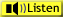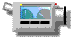ChaptersThis text is meant to accompany class discussions. It is not everything there is to know about uniform circular motion. It is meant as a  prep for class. More detailed notes and examples are given in the class notes, presentations, and demonstrations (click here.)

Implied Givens and More About Projectile Motion

"Apogee"is defined as the highest part of the projectile's motion where the vertical component of velocity is equal to zero. The horizontal velocity is not zero, only the vertical velocity.The arrows in the drawing above are the components of the velocity for each position. Notice how the horizontal velocity is the same length and direction for every position.

This piece of information implies the final vertical velocity is zero in the givens table. Below is an example of where the information about apogee is placed.When a question asks about apogee you may need to create a temporary table of givens where the givens reflect what is known about apogee as shown above and in the example below.

 Example 7 Calculations to Apogee

Example 8 ...More Calculations to Apogee
 A spear is thrown off of a high ledge with the givens shown in the picture to the right. How much time does it take to get to apogee? (Neglect the height of the person throwing the spear.)SolutionHere is a YouTube video showing the solution below, http://youtu.be/_YqpjaCiRxo. It will open in a new window.

More Apogee Concepts to Keep in MindWhen landing at the same height as the initial velocity;

• the time to apogee is equal to the time from apogee,
• the initial velocity is equal to the negative of the final velocity,
• the distance to apogee is equal to the distance from apogee.

More Concepts to Help Define the Givens

When a projectile lands at the same height it is launched from the initial velocity is equal in magnitude to the impact velocity and in the opposite direction.

The two pulsating projectiles have equal and opposite velocities. For a problem where the projectile lands at the same height it is launched from

• The launch velocity is equal and opposite to the impact velocity.
• The time from the ground up to apogee is equal to the time from apogee to the ground.
• The distance from the ground to apogee is equal to the distance from apogee to the ground.
• When solving for the total time of flight from launch to impact, the displacement Δx = 0. (This is because it lands at the same height it is launched from.

In terms of the list of givens this means that the the magnitude of vimpact = –vo.

It also means when finding the time to apogee, the problem could be reversed and the projectile could be dropped from apogee. This could be handy because it would make the initial velocity equal to zero.

Initial Velocities that are Horizontal

Some projectile problems involve a ball rolling off a ledge horizontally.

In this situation the horizontal component of the initial velocity is the same as the initial velocity. The vertical initial velocity is zero. In the steps outlined earlier, skip step number one -where a triangle is drawn. The data table for the animation above would look like the one below.

 vertical horizontal vo 0 5 m/s x t v a -9.80m/s2 0
 Example 9 ...What to do when Vo is horizontal A dog runs horizontally off a ledge 3.00 m above a pond. The dog was running at 6.00 m/s. How far away from the edge of the ledge did the dog land? (Looking for the range.) Solution

by Tony Wayne ...(If you are a teacher, please feel free to use these resources in your teaching.)